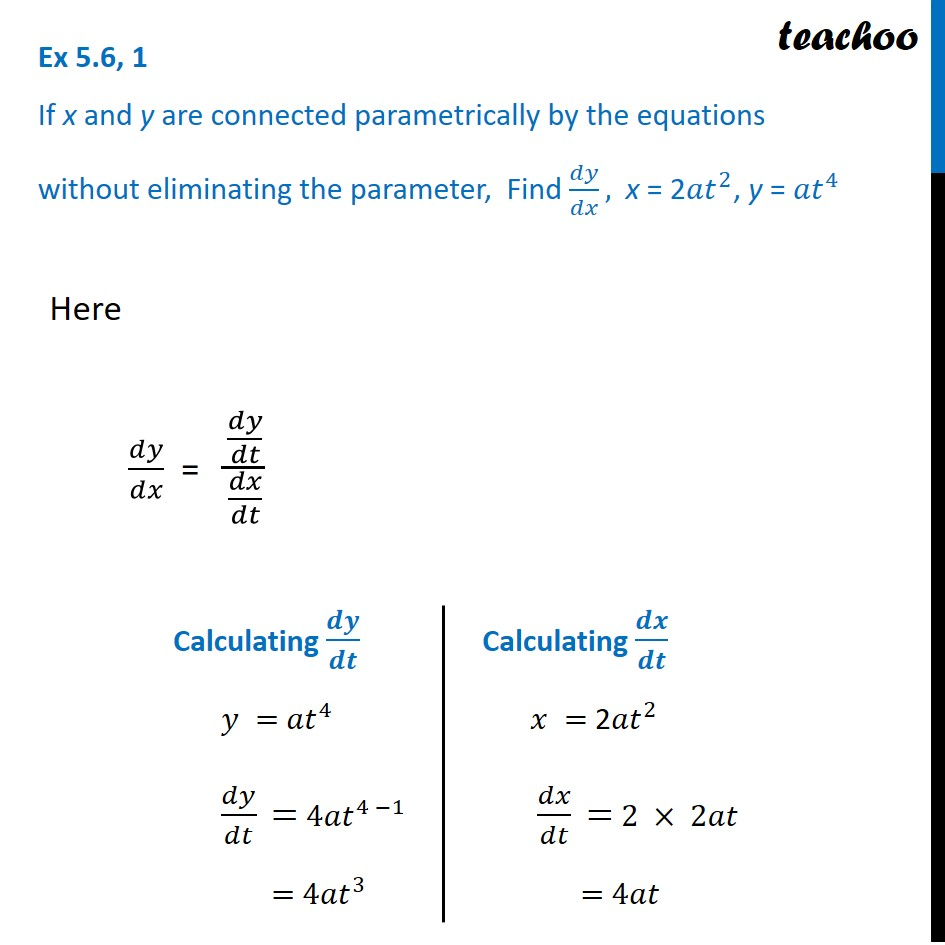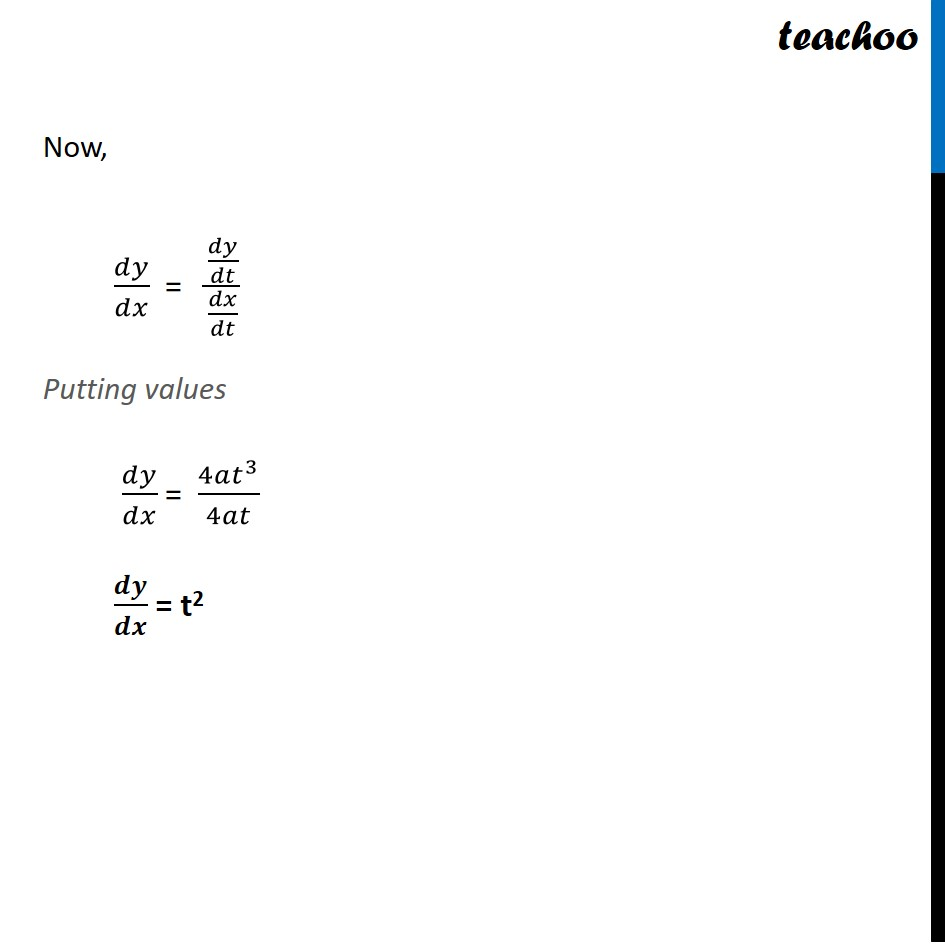Ex 5.6

Chapter 5 Class 12 Continuity and Differentiability
Serial order wiseLearn in your speed, with individual attention - Teachoo Maths 1-on-1 Class

### Transcript

Ex 5.6, 1 If x and y are connected parametrically by the equations without eliminating the parameter, Find 𝑑𝑦/𝑑𝑥, x = 2〖𝑎𝑡〗^2, y = 〖𝑎𝑡〗^4Here 𝑑𝑦/𝑑𝑥 = (𝑑𝑦/𝑑𝑡)/(𝑑𝑥/𝑑𝑡) Calculating 𝒅𝒚/𝒅𝒕 𝑦 = 〖𝑎𝑡〗^4 𝑑𝑦/𝑑𝑡 " =" 〖4𝑎𝑡〗^(4 −1) "=" 〖4𝑎𝑡〗^3 Calculating 𝒅𝒙/𝒅𝒕 𝑥 = 2〖𝑎𝑡〗^2 𝑑𝑥/𝑑𝑡 " =" 2 × 2𝑎𝑡 "=" 4𝑎𝑡 Now, 𝑑𝑦/𝑑𝑥 = (𝑑𝑦/𝑑𝑡)/(𝑑𝑥/𝑑𝑡) Putting values 𝑑𝑦/𝑑𝑥 = (4𝑎𝑡^3)/4𝑎𝑡 𝒅𝒚/𝒅𝒙 = t2